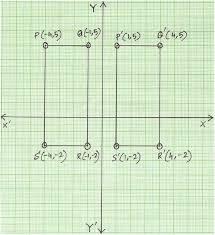# Determine 8134

The perimeter of the rectangle that can be divided into three squares is 168 cm. Determine the lengths of its sides.

a =  63 cm
b =  21 cm

### Step-by-step explanation:

2(a+b) = 168
a = 3b

2·(a+b) = 168
a = 3·b

2a+2b = 168
a-3b = 0

Row 2 - 1/2 · Row 1 → Row 2
2a+2b = 168
-4b = -84

b = -84/-4 = 21
a = 168-2b/2 = 168-2 · 21/2 = 63

a = 63
b = 21

Our linear equations calculator calculates it.Did you find an error or inaccuracy? Feel free to write us. Thank you!

Tips for related online calculators
Do you have a linear equation or system of equations and looking for its solution? Or do you have a quadratic equation?
Do you want to convert length units?

#### Grade of the word problem:

We encourage you to watch this tutorial video on this math problem: